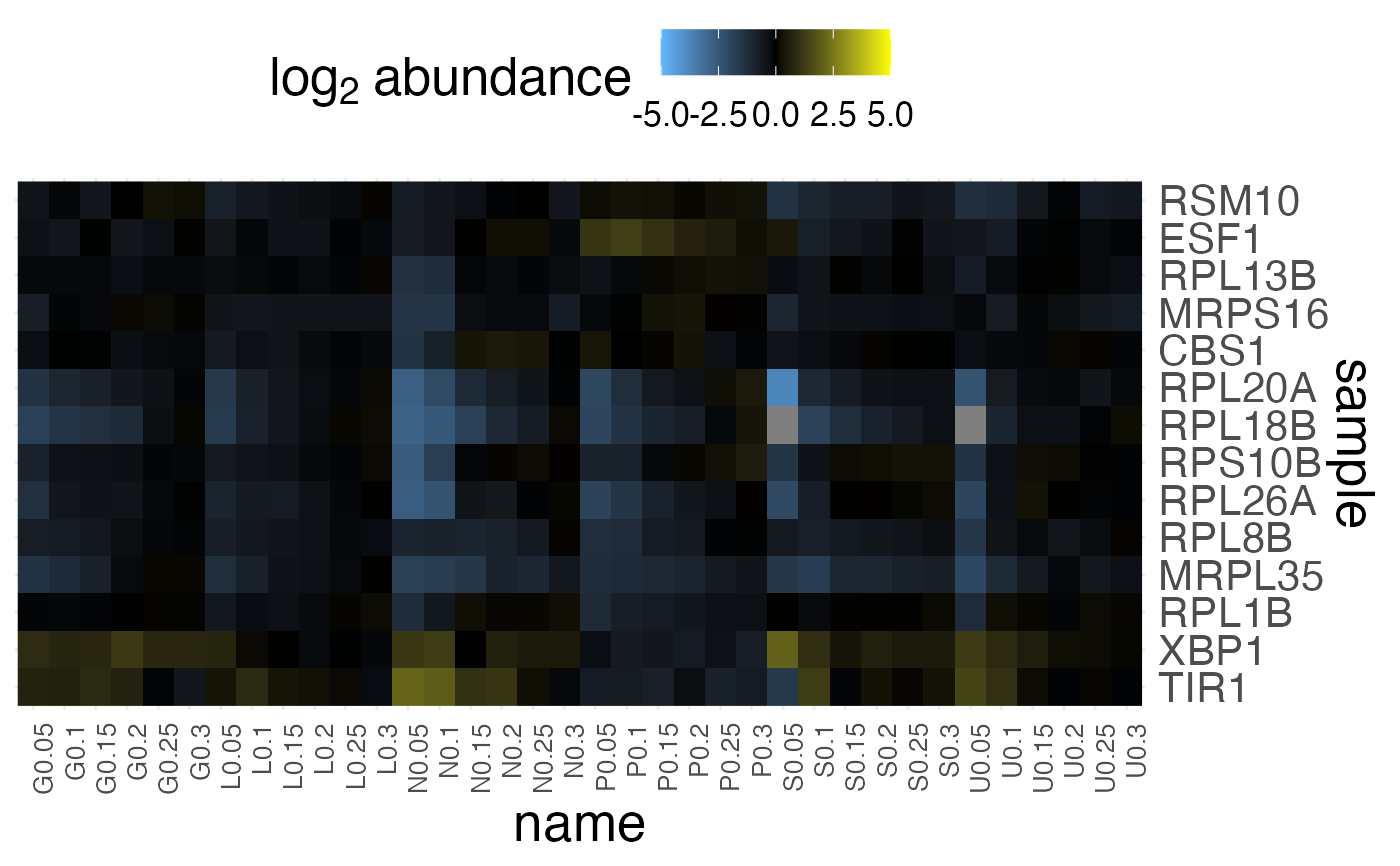Generate a heatmap visualization of a features x samples matrix of measurements.

plot_heatmap(
tomic,
feature_var = NULL,
sample_var = NULL,
value_var = NULL,
cluster_dim = "both",
distance_measure = "dist",
hclust_method = "ward.D2",
change_threshold = Inf,
plot_type = "grob",
max_display_features = 800
)

## Arguments

tomic

Either a tidy_omic or triple_omic object

feature_var

variable from "features" to use as a unique feature label.

sample_var

variable from "samples" to use as a unique sample label.

value_var

which variable in "measurements" to use for quantification.

cluster_dim

rows, columns, or both

distance_measure

variable to use for computing dis-similarity

corr

pearson correlation

dist

euclidean distance

hclust_method

method from stats::hclust to use for clustering

change_threshold

values with a more extreme absolute change will be thresholded to this value.

plot_type

plotly (for interactivity) or grob (for a static ggplot)

max_display_features

aggregate and downsample distinct feature to this number to speed to up heatmap rendering.

a ggplot2 grob

## Examples


library(dplyr)

tomic <- brauer_2008_triple %>%
filter_tomic(
filter_type = "category",
filter_table = "features",
filter_variable = "BP",
filter_value = c(
"protein biosynthesis",
"rRNA processing", "response to stress"
)
)

plot_heatmap(
tomic = tomic,
value_var = "expression",
change_threshold = 5,
cluster_dim = "rows",
plot_type = "grob",
distance_measure = "corr"
)